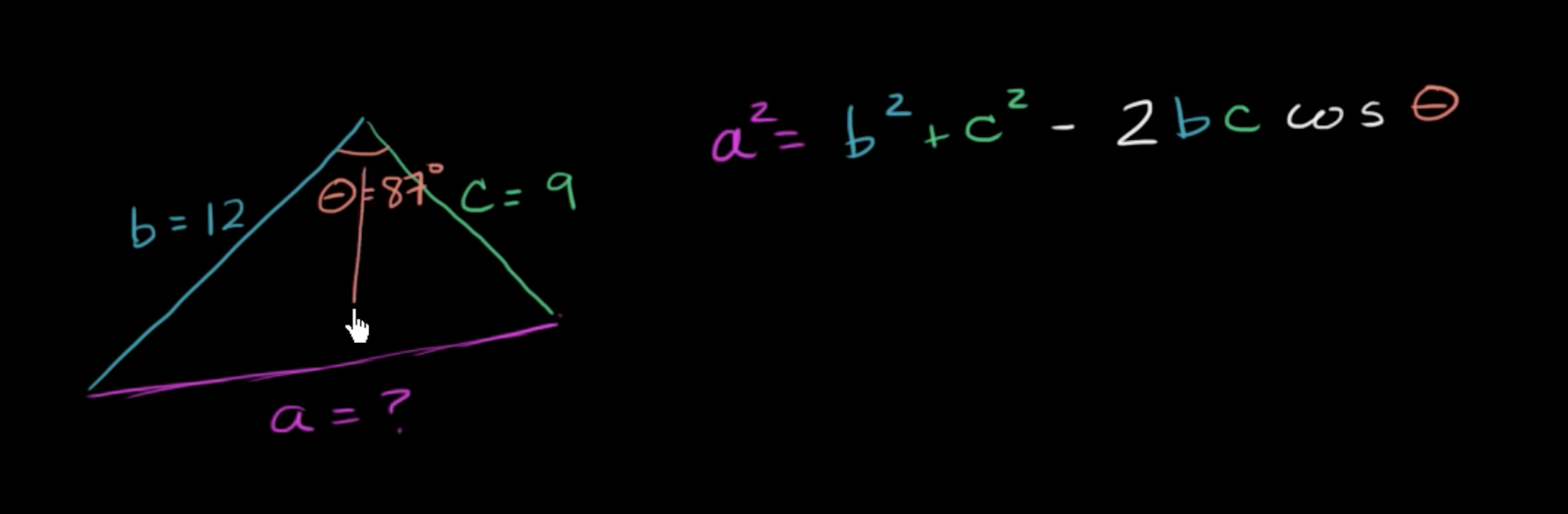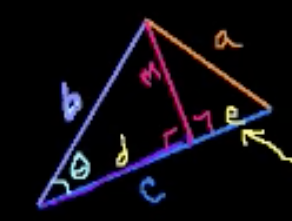## Law of cosines (Khan Academy)These are notes from the Khan Academy video series on Law of Cosines.

## Solving for a side with the law of cosines

Given a triangle with sides A, B, and C and angle AB, if you know the length of A and B and angle AB, how can you calculate C?

If the triangle is a right-angle triangle, we can solve a missing side using Pythagoras Theorem:

$C^2 = B^2 + A^2$

For any triangle, we can use the law of cosines:

$C^2 = B^2 + A^2 - 2AB \cdot \cos(\theta)$

This expression makes sense because $\cos(90°) = 0$ . For a right-angle triangle, you can simply ignore the $-2AB \cdot \cos(\theta)$ component.

## Solving for an angle with the law of cosines

If the angle is unknown, we can rewrite the expression as follows:

$cos(C) = \frac{a^2 + b^2 - c^2}{2ab}$

or

$C = acos(\frac{a^2 + b^2 - c^2}{2ab})$

## Proof of the law of cosines

One way to solve the problem using the law of cosines is to convert them into two right triangles.Then we can calculate the side $d$ knowing CAH: Cosine =Adjacent / Hypotenuse.

$\cos(\theta)=\frac{d}{b} \Rightarrow d = b\cos(\theta)$

We know that $e$ is $e = c - b\cos(\theta)$

Can calculate side $m$ since $m$ is opposite, we can use SOH: Sine = Opposite / Hypotenuse.

$\frac{m}{b}=\sin\theta \Rightarrow m=b \sin(\theta)$

Now we can use Pythagorean formula:

$a^2 = m^2 + e^2$ $a^2 = (b\sin(\theta))^2 + (c-b\cos(\theta))^2$

We can then multiply it out:

$b^2sin^2 \theta + c^2 - 2cb \cos\theta + b^2\cos^2 \theta$

We can then rearrange the expression:

$b^2 sin^2 \theta + b^2 \cos^2 \theta + c^2 - 2bc \cos \theta$

Which can be rewritten as:

$b^2(\sin^2\theta + \cos^2 \theta) + c^2 -2bc \cos \theta$

Since the first part of the expression is the product of $b^2$ and the Pythagorean identity, we can reduce it to $b^2$

$a^2 = b^2 + c^2 -2bc \cos \theta$

And that's the formula for the law of cosines!